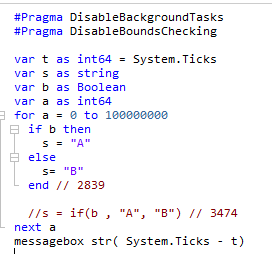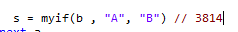# If() and if..then speed differences

Is there any speed difference when doing:

``````var t as string
t = if(<expression>, "1","2")
``````

and

``````var t as string
if <expression> then
t = "1"
else
t = "2"
end
``````

Time 1 million laps of your question, let’s see it.

I know. But maybe someone knows the answer (and reason) already.Not sure about Xojo, but DEPENDING on the context and involved code, the first code, IF solved by an smart compiler using registers efficiently, COULD be faster. But, depending on how things are solved, maybe both could end with practically the same code.

Maybe this one is faster``````var t as string = if(<expression>, "1","2")
``````
1 Like

The way to know is just measuring it or disassembling it to see how it solves both.

The single line if is a bit like tenary operator. Which usually is tiny bit faster than the other way. But there is no way to know without testing how it ends up compiling and optimizing in Xojo.

Well, it is a lot slower. Did the following test:

var t as int64 = System.Ticks
var t as string
var b as Boolean
var a as int64
for a = 0 to 100000000
'if b = true then
't = “A”
'else
't=“B”
'end

t = if(b , “A”, “B”)
next a
messagebox str( System.Ticks - t)

With If…then it took approx 729 ticks
With If() it took approx 1207ticks.

So there you go … it is about double slower.

2 Likes

This is a function:

`If()`

This is not:

``````If .. Then
// Do something
End If
``````

We all could have hoped Xojo didn’t just add an “If” function and instead added a short if statement (operators?) that’s actually optimized. Lot’s of things in Xojo could have been optimized.
This does give everone a view of the speed for these kind of things, which is nice actually.

Did the same test with Swift.
And with Swift it is about double FASTER.
So Ternary Operators are much faster in Swift (as it is expected).
My guess is that Xojo doesn’t really compiles it as a ternary operator. A bit of a fake way to use if() :-/

1 Like

Well in swift it’s an operator, just as in c/c++ it would be.

Maybe you can:

``````Var myBool As Boolean = true
Var s As String = ""
If myBool Then s = "YES" else s = "NOPE"
``````

This is an expression. A Meta-function. Such expression could be solved optimized inline, or using some lazy way, like emulating its solution using already available “engines” like creating and calling a real function behind of scenes.

You are right the in place if is suspiciously slow to say the least:And as Rick says their probably implementing it internally as function which could explain the difference, since then you have just the same as the other case + the overhead of calling a function.

No making it a function makes it even slower:So the internal is maybe not function.

And when you hoover over it it claims to be operator. And also if it was a function you would run into trouble with return type if your doing it on a special type like class of type Guest. (while operator as it claims to be would resolve that correctly as it does)

Also if it were a function, the Else clause would be evaluated even when the condition is True, and it is not.

Compiled or within the IDE? If compiled, what optimization level?

My quick test was just in IDE

Yes it claims to be:
https://docs.xojo.com/If

Then it’s for sure in no way optimized, maybe it could have just converted that code into a true if statement so it’s just a convient way to type it.

Compiled Aggressive optimization it goes to:

50 (for normal if then else)
and 2036 (for in place if)

I am assuming where it goes to 50 it just decides that the whole loop does nothing, while in the later case it does not realize that.

So not really good test I guess.

``````Private Function myIf(condition As Boolean, trueValue As Variant, falseValue As Variant) As Variant
If condition Then
Return trueValue
End If

Return falseValue
End Function
``````

like that, where the first condition is always evaluated but the last is only when the first is false?
The variants may be a bad example…

Forum for Xojo Programming Language and IDE. Copyright © 2021 Xojo, Inc.